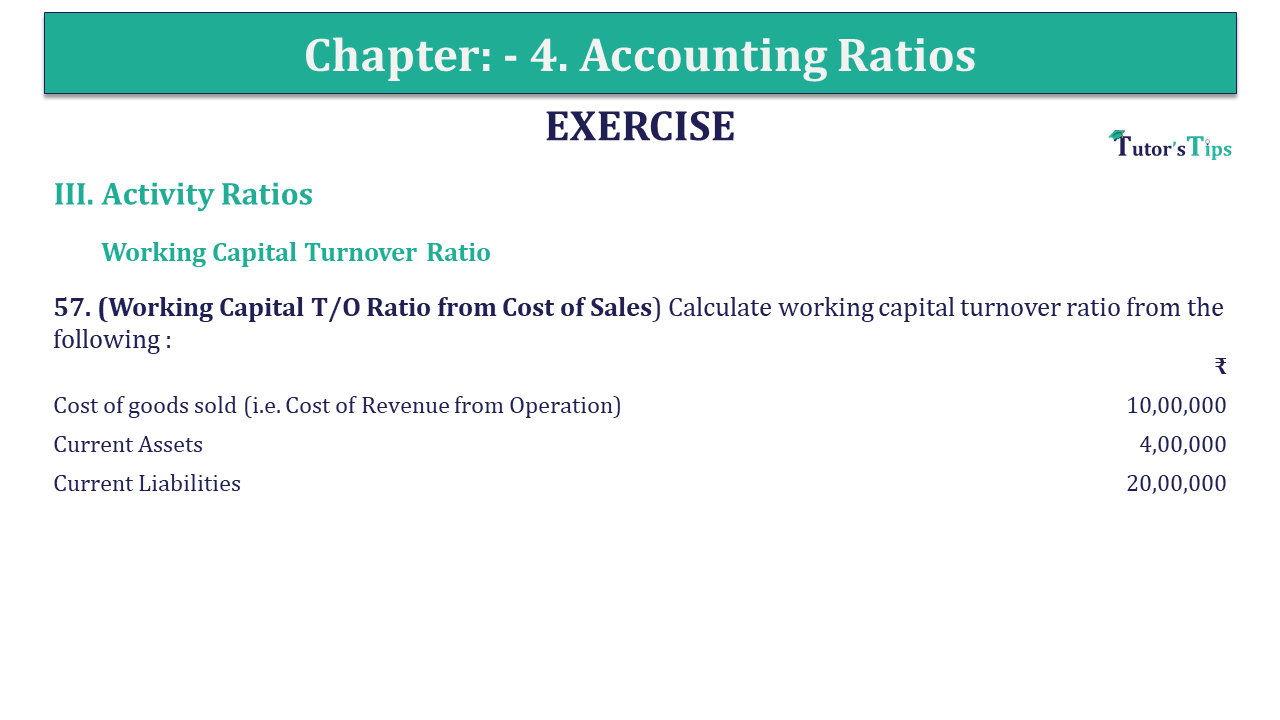# Question 57 Chapter 4 of +2-B – USHA Publication 12 ClassQ-57- CH-4 Book 2 - Usha Pub. +2 Book 2020 - Solution

Question 57 Chapter 4 of +2-B

Working Capital Turnover Ratio

57. (Working Capital T/O Ratio from Cost of Sales) Calculate working capital turnover ratio from the following :

 ₹ Cost of goods sold (i.e. Cost of Revenue from Operation) 10,00,000 Current Assets 4,00,000 Current Liabilities 20,00,000

## The solution of Question 57 Chapter 4 of +2-B: –

 Working Capital Turnover Ratio = Sales or Revenue from Operation Working Capital Cost of Revenue from Operation = ₹ 2,00,000 Working Capital = Current Assets – Current Liabilities = ₹ 1,00,000 – ₹ 50,000 = ₹ 50,000 = ₹ 2,00,000 Working Capital Turnover Ratio ₹ 5,00,000 = 4 times

*In this question sales or revenue from an operation is not given, to solve this question Cost of Revenue from Operation are taken for the calculation of the ratio.

Also, Check out the solved question of previous Chapters: –